# Python Tuples

Tuples in Python are a type of sequence just like strings. But in case of tuple, it can contain element of any type.

You can use tuple in python to store:

• group of names, for example, employee names, or student names etc.
• and bunch of high scores etc.

Tuples can not be changed unlike the lists and tuples uses parentheses.

Note - Tuple elements can be of one or more than one types.

You can create a tuple in python with both strings and numbers. And can also create a sequence of graphics, images files, or audio files etc.

A tuple is basically a sequence of immutable python objects.

## Create an empty Tuple in Python

Here is an example shows how to create an empty tuple in python.

`my_tuple = ();`

As you can see from the above general form to create an empty tuple in python, the variable named my_tuple is the name of the tuple.

## Create a Tuple with Items in Python

Now let's see how to create a tuple with some items in python with the help of following general form.

`my_tuple = ("me", "my friend", "my brother", "my sister");`

Here is another example to create tuples in python with some items:

```tuple1 = ("python", "tuple", 1952, 2323, 432);
tuple2 = (1, 2, 3, 4, 5);
tuple3 = ("a", "b", "c", "d", "e");```

## Python Tuple Example

Let's take an example to understand about tuple in better way.

```# Python Tuple Example
print("Creating an empty tuple...");
my_tuple = ();
print("An empty tuple, my_tuple is created successfully.");
if not my_tuple:
print("The tuple, my_tuple, contains no any item.");
print("Inserting some items to the tuple...");
my_tuple = ("me", "my friend", "my brother", "my sister");
print("\nPrinting the tuple...");
print(my_tuple);
print("\nNow printing each item in the tuple...");
for item_in_tuple in my_tuple:
print(item_in_tuple);```

Here is the screenshot of the sample output produced by the above example of tuple in python.Here is an example program, illustrating the tuple in Python:

```# Python Tuple - Example Program

tuple1 = ("python", "tuple", 1952, 2323, 432);

print(tuple1)              # this will print the complete tuple
print(tuple1[1:4])         # this will print the elements starting from 2nd till 4th
print(tuple1[1:])          # this will print the elements starting from the 2nd element
print(tuple1)           # this wil print the first element of the tuple
print(tuple1 * 2)          # this will print the tuple two times```

Here is the sample output of the above python program:A tuple containing single value also have a comma like this

`tuple1 = (10,);`

Let's take another example of tuple in python. This is a simple example of tuple.

```# Python Tuple Example
my_tuple = ("zero", "one", "two", "three", "four");
print("Items in the tuple, my_tuple is:");
for t in my_tuple:
print(t);```

If you run the above tuple example code, then below is the sample output you will see on your screen: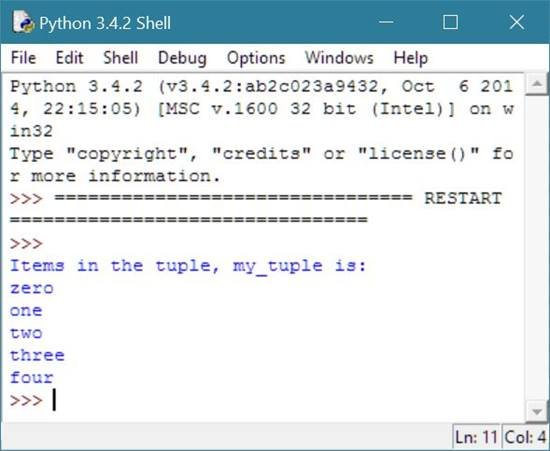As you can see from the above example, the tuple my_tuple is created and initialized some items to it, and then prints all the items using python for loop.

## Find Length of Tuple in Python

The len() function in python is used to find the length of the tuple as shown in the following example code.

```# Finding length of a tuple example in python
my_tuple = ("zero", "one", "two", "three", "four");
length_of_tuple = len(my_tuple);
print("Length of the tuple, my_tuple is:",length_of_tuple);```

Here is the sample output produced by the above example code of finding length of a tuple.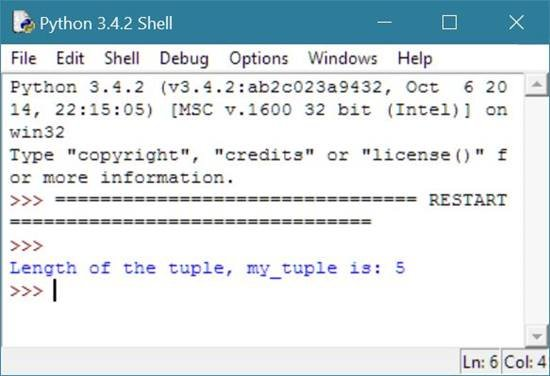## Check Element Present in Tuple or Not

You can use in operator to check whether a particular element or item is present inside a tuple or not in python as shown in the example given below.

```# check if an element is present inside a tuple or not
my_tuple = ("zero", "one", "two", "three", "four");
if "one" in my_tuple:
print("'one' is present as an element inside the tuple, my_tuple.");
else:

Here is the sample output produced by the above example of checking whether an element present inside a particular tuple or not. As you can see from the above example, one is present inside the tuple, my_tuple as its item, therefore the statement of if will be executed, that is, shown in below screenshot:## Indexing Tuple in Python

Indexing tuple in python means, finding the exact position of an element or item present inside the tuple to access that element to perform the action, like adding, displaying, replacing, removing etc.

Here is an example shows how to index an element in the tuple.

```# Indexing tuple in python to find the position of an element
my_tuple = ("zero", "one", "two", "three", "four");
index_no = input("Enter index number of the element:");
index_no = int(index_no);
print(my_tuple[index_no],"is present inside the tuple at this index number.");```

Here is the sample output (initial output) produced by the above indexing tuple example code in python: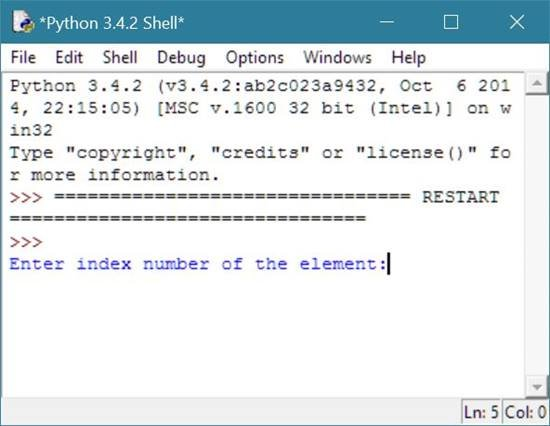Now provide the index number, say 1, and press enter to watch the follwing output: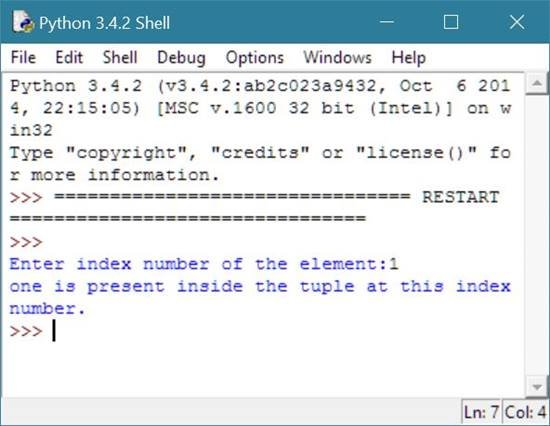Note - Index starts from 0.

## Slicing Tuple in Python

Slicing items or elements from a tuple in python, means extracting or taking elements from the tuple by providing starting or beginning and ending index number of the tuple.

In other word, to slice tuple in python, you have to provide the starting and ending index number to slice all the items present between these two index numbers from the tuple in python as shown in the example given below.

```# Slicing tuple in python example
my_tuple = ("zero", "one", "two", "three", "four");
starting_index_no = input("Enter starting index no.:");
ending_index_no = input("Enter ending index no.:");
starting_index_no = int(starting_index_no);
ending_index_no = int(ending_index_no);
print("\nSlicing elements from the tuple....\n");
print("Here are the items sliced from the tuple according to your given index numbers:");
print(my_tuple[starting_index_no:ending_index_no]);```

Here is the sample output of the above slicing tuple example code in python. This is the initial output:Now provide starting and ending index number, say 2 and 5 and press ENTER key to see the following output: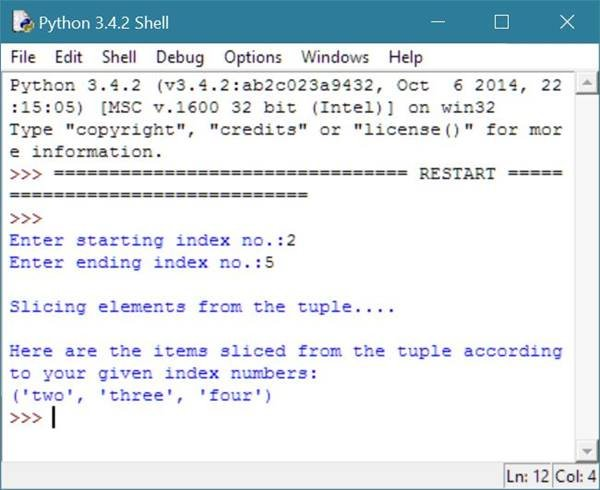## Concatenating Tuples in Python

Concatenating tuples in python means adding one tuple into another or you can say concatenating tuples means joining them together with the help of plus (+) operator available in python as shown in the example given below.

```# Simple concatenation of tuple example in python
my_tuple = ("zero", "one", "two", "three", "four");
my_new_tuple = ("five", "six", "seven");
my_tuple += my_new_tuple;
print("Tuple after concatenating both the tuple:");
for mt in my_tuple:
print(mt);```

Here is the sample output produced by the above concatenating tuple example in python.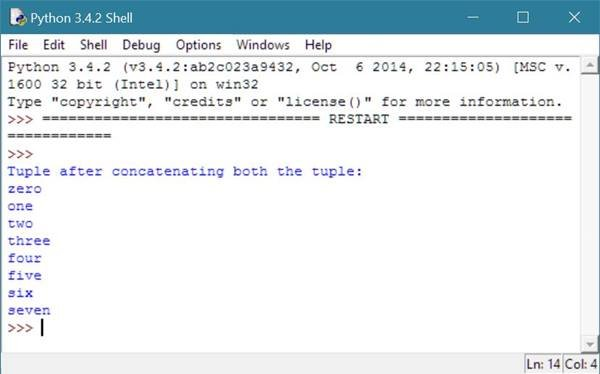Now let's modify the above tuple concatenation example code in python.

```# Concatenating tuple in python
my_tuple = ("zero", "one", "two", "three", "four");
my_new_tuple = ("five", "six", "seven");
print("Elements in my_tuple:");
for mt in my_tuple:
print(mt);
print("\nElements in my_new_tuple:");
for mnt in my_new_tuple:
print(mnt);
print("\nConcatenating both the tuple....");
my_tuple += my_new_tuple;
print("\nTuple after concatenating both the tuple:");
for mt in my_tuple:
print(mt);```

Now this time, you will see the following output on your screen after executing the above example code of tuple concatenation in python.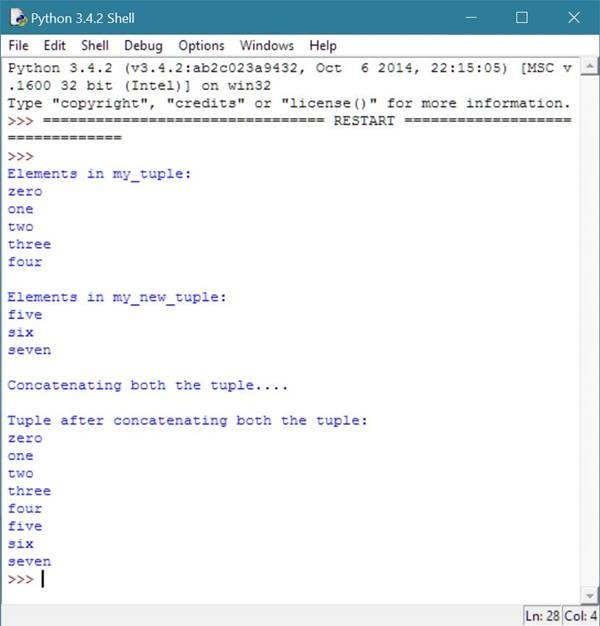Tools
Calculator